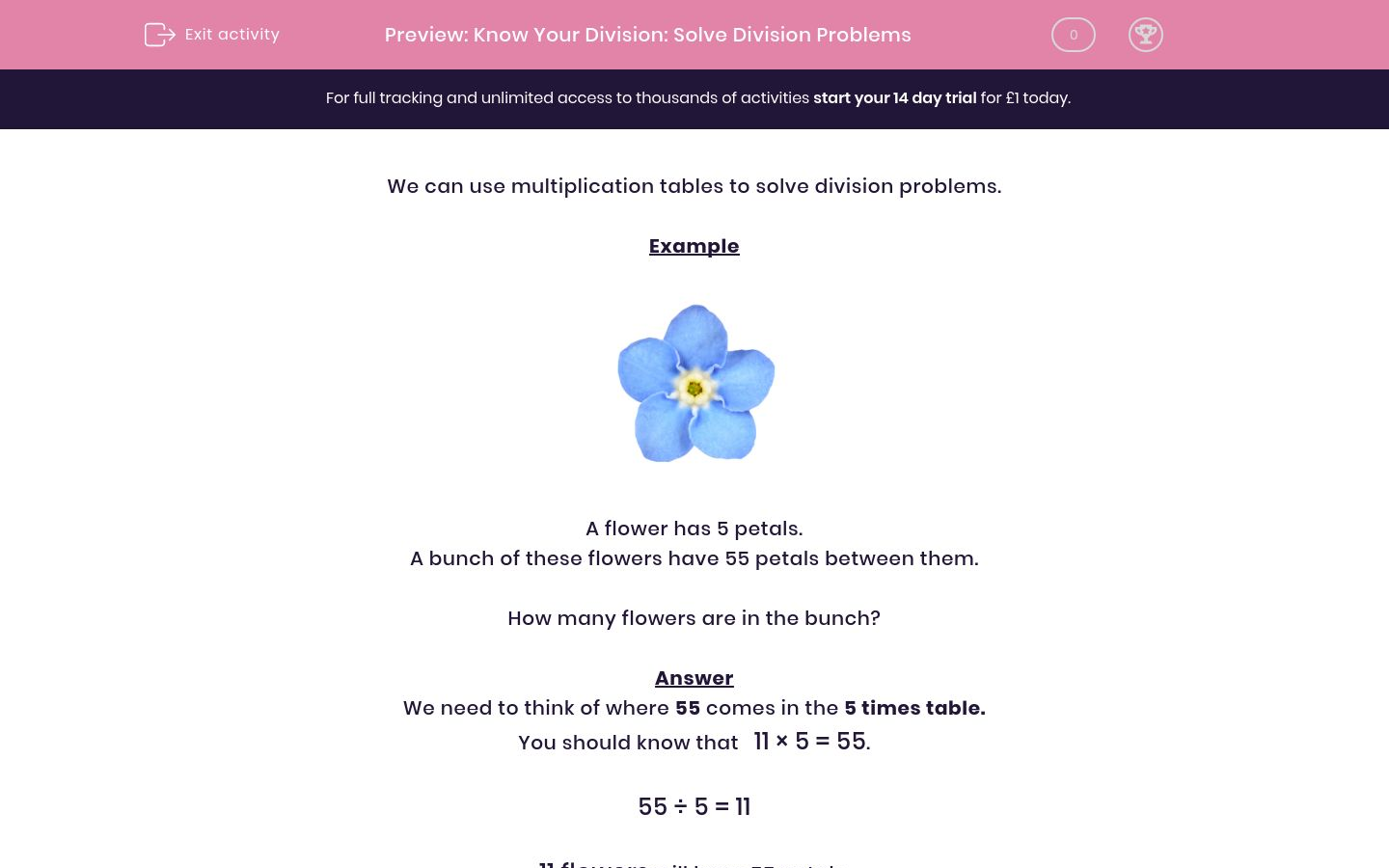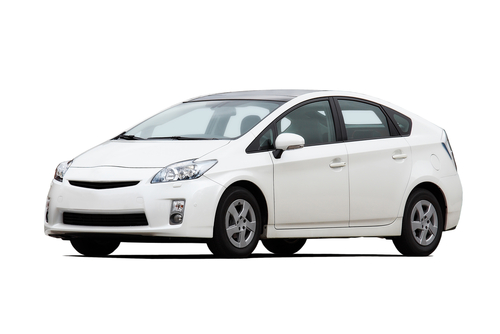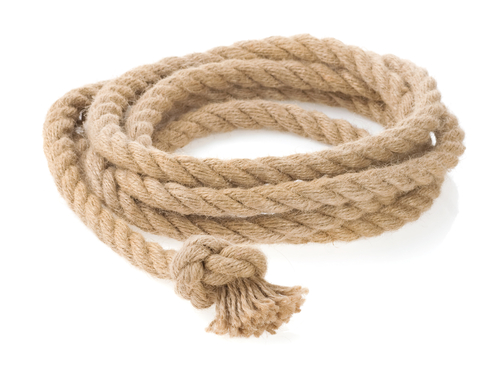# Know Your Division: Solve Division Problems

In this worksheet, students must solve division problems using multiplication tables.Key stage:  KS 2

Curriculum topic:   Maths and Numerical Reasoning

Curriculum subtopic:   Word Problems

Difficulty level:### QUESTION 1 of 10

We can use multiplication tables to solve division problems.

ExampleA flower has 5 petals.

A bunch of these flowers have 55 petals between them.

How many flowers are in the bunch?

We need to think of where 55 comes in the 5 times table.

You should know that   11 × 5 = 55.

55 ÷ 5 = 11

11 flowers will have 55 petals.8 friends are going to the cinema.

Their 8 cinema tickets cost £48 in total.

How much does each ticket cost in pounds?

(Just write the number.)A star has 5 points.

A group of these stars has 60 points altogether.

How many stars are in the group?An orchard has 8 rows of trees.

There are 64 trees in total.

Use division to work out how many trees are in each row.A car has 4 wheels.

In a car park, I count 36 wheels on all the cars.

How many cars are in the car park?

4 children collected £32 for charity.

They each collected the same amount.

How much did they each collect in pounds?

(Just write the number.)Apples cost 8p each.

I spend 72p on apples.

How many apples do I buy?A piece of rope is 96 m long.

John cuts it into pieces which are each 8 metres long.

How many pieces of rope does he now have?This flower has 6 petals.

A bunch of these flowers has 42 petals in total.

How many flowers are in the bunch?

Fill in the missing number:

28  ÷=

Fill in the missing number:

88  ÷=  8

• Question 18 friends are going to the cinema.

Their 8 cinema tickets cost £48 in total.

How much does each ticket cost in pounds?

(Just write the number.)

6
EDDIE SAYS
6 × 8 = 48
48 ÷ 8 = £6
• Question 2A star has 5 points.

A group of these stars has 60 points altogether.

How many stars are in the group?

12
EDDIE SAYS
12 × 5 = 60 points
60 ÷ 5 = 12 stars
• Question 3An orchard has 8 rows of trees.

There are 64 trees in total.

Use division to work out how many trees are in each row.

8
EDDIE SAYS
8 × 8 = 64 trees
64 ÷ 8 = 8 trees in each row.
• Question 4A car has 4 wheels.

In a car park, I count 36 wheels on all the cars.

How many cars are in the car park?

9
EDDIE SAYS
9 × 4 = 36 wheels
36 ÷ 4 = 9 cars
• Question 5

4 children collected £32 for charity.

They each collected the same amount.

How much did they each collect in pounds?

(Just write the number.)

8
EDDIE SAYS
8 × 4 = 32
32 ÷ 4 = £8
• Question 6Apples cost 8p each.

I spend 72p on apples.

How many apples do I buy?

9
EDDIE SAYS
9 × 8 = 72p
72 ÷ 8 = 9 apples
• Question 7A piece of rope is 96 m long.

John cuts it into pieces which are each 8 metres long.

How many pieces of rope does he now have?

12
EDDIE SAYS
12 × 8 = 96 metres
96 ÷ 8 = 12 pieces
• Question 8This flower has 6 petals.

A bunch of these flowers has 42 petals in total.

How many flowers are in the bunch?

7
EDDIE SAYS
7 × 6 = 42 petals
42 ÷ 6 = 7 flowers
• Question 9

Fill in the missing number:

28  ÷=

4
EDDIE SAYS
4 × 7 = 28
28 ÷ 4 = 7
• Question 10

Fill in the missing number:

88  ÷=  8

11
EDDIE SAYS
8 × 11 = 88
88 ÷ 11 = 8
---- OR ----

Sign up for a £1 trial so you can track and measure your child's progress on this activity.

### What is EdPlace?

We're your National Curriculum aligned online education content provider helping each child succeed in English, maths and science from year 1 to GCSE. With an EdPlace account you’ll be able to track and measure progress, helping each child achieve their best. We build confidence and attainment by personalising each child’s learning at a level that suits them.

Get started